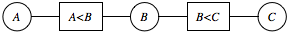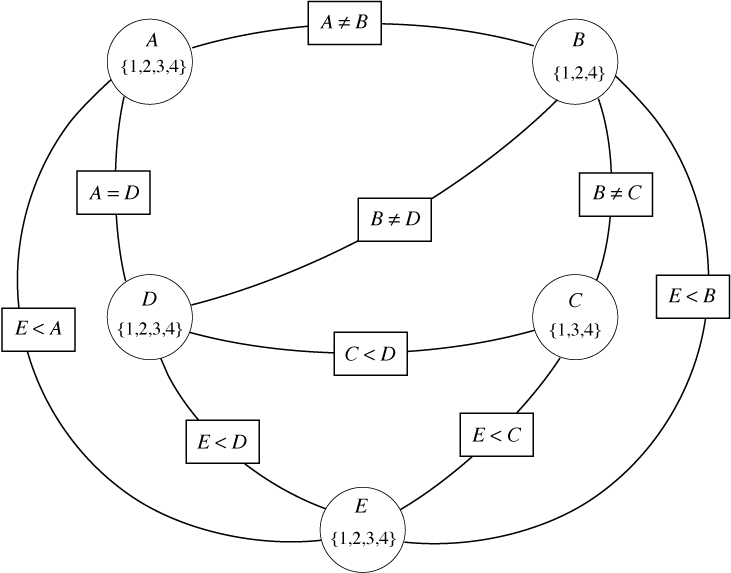## 4.5 Consistency Algorithms

Although depth-first search over the search space of assignments is usually a substantial improvement over generate and test, it still has various inefficiencies that can be overcome.

Example 4.14: In Example 4.13, the variables A and B are related by the constraint A<B. The assignment A=4 is inconsistent with each of the possible assignments to B because dom(B)={1,2,3,4}. In the course of the backtrack search (see Figure 4.1), this fact is rediscovered for different assignments to B and C. This inefficiency can be avoided by the simple expedient of deleting 4 from dom(A), once and for all. This idea is the basis for the consistency algorithms.

The consistency algorithms are best thought of as operating over the network of constraints formed by the CSP:

• There is a node for each variable. These nodes are drawn as ovals.
• There is a node for each constraint. These nodes are drawn as rectangles.
• Associated with each variable, X, is a set DX of possible values. This set of values is initially the domain of the variable.
• For every constraint c, and for every variable X in the scope of c, there is an arc ⟨X,c⟩.

Such a network is called a constraint network.Figure 4.2: Constraint network for the CSP of Example 4.15

Example 4.15: Consider Example 4.13. There are three variables A, B, and C, each with domain {1,2,3,4}. The constraints are A<B and B<C. In the constraint network, shown in Figure 4.2, there are four arcs:
 ⟨A,A
Example 4.16: The constraint X≠4 has one arc:
 ⟨X,X≠4⟩

The constraint X+Y=Z has three arcs:

 ⟨X,X+Y=Z⟩ ⟨Y,X+Y=Z⟩ ⟨Z,X+Y=Z⟩

The simplest case is when the constraint has just one variable in its scope. In this case, the arc is domain consistent if every value of the variable satisfies the constraint.

Example 4.17: The constraint B≠3 has scope {B}. With this constraint, and with DB={1,2,3,4}, the arc ⟨B, B≠3⟩ is not domain consistent because B=3 violates the constraint. If the value 3 were removed from the domain of B, then it would be domain consistent.

Suppose constraint c has scope {X,Y1,...,Yk}. Arc ⟨X,c⟩ is arc consistent if, for each value x∈DX, there are values y1,...,yk where yiDYi, such that c(X=x,Y1=y1,...,Yk=yk) is satisfied. A network is arc consistent if all its arcs are arc consistent.

Example 4.18: Consider the network of Example 4.15. None of the arcs are arc consistent. The first arc is not arc consistent because for A=4 there is no corresponding value for B for which A<B. If 4 were removed from the domain of A, then it would be arc consistent. The second arc is not arc consistent because there is no corresponding value for A when B=1.

If an arc ⟨X,c⟩ is not arc consistent, there are some values of X for which there are no values for Y1,...,Yk for which the constraint holds. In this case, all values of X in DX for which there are no corresponding values for the other variables can be deleted from DX to make the arc ⟨X,c⟩ consistent.

1: Procedure GAC(V,dom,C)
2:           Inputs
3:                     V: a set of variables
4:                     dom: a function such that dom(X) is the domain of variable X
5:                     C: set of constraints to be satisfied
6:           Output
7:                     arc-consistent domains for each variable
8:           Local
9:                     DX is a set of values for each variable X
10:                     TDA is a set of arcs
11:           for each variable X do
12:                     DX ←dom(X)
13:           TDA ←{⟨X,c⟩| c∈C  and X∈scope(c)}
14:           while (TDA ≠{})
15:                     select ⟨X,c⟩ ∈TDA;
16:                     TDA ←TDA  \ {⟨X,c⟩};
17:                     NDX ←{x| x ∈DX and some {X=x,Y1=y1,...,Yk=yk}∈c where yiDYi for all i}
18:                     if (NDXDX) then
19:                               TDA ←TDA ∪{⟨Z,c'⟩|X ∈scope(c'), c' is not c, Z∈scope(c') \ {X} }
20:                               DX ←NDX
21:           return {DX| X  is a variable}
Figure 4.3: Generalized arc consistency algorithm

The generalized arc consistency algorithm is given in Figure 4.3. It makes the entire network arc consistent by considering a set of potentially inconsistent arcs, the to-do arcs, in the set TDA. TDA initially consists of all the arcs in the graph. While the set is not empty, an arc ⟨X,c⟩ is removed from the set and considered. If the arc is not consistent, it is made consistent by pruning the domain of variable X. All of the previously consistent arcs that could, as a result of pruning X, have become inconsistent are placed back into the set TDA. These are the arcs ⟨Z,c'⟩, where c' is a constraint different from c that involves X, and Z is a variable involved in c' other than X.

Example 4.19: Consider the algorithm GAC operating on the network from Example 4.15. Initially, all of the arcs are in the TDA set. Here is one possible sequence of selections of arcs:
• Suppose the algorithm first selects the arc ⟨A,A<B⟩. For A=4, there is no value of B that satisfies the constraint. Thus, 4 is pruned from the domain of A. Nothing is added to TDA because there is no other arc currently outside TDA.
• Suppose that ⟨B,A<B⟩ is selected next. The value 1 can be pruned from the domain of B. Again no element is added to TDA.
• Suppose that ⟨B,B<C⟩ is selected next. The value 4 can be removed from the domain of B. Because the domain of B has been reduced, the arc ⟨A,A<B⟩ must be added back into the TDA set because the domain of A could potentially be reduced further now that the domain of B is smaller.
• If the arc ⟨A,A<B⟩ is selected next, the value A=3 can be pruned from the domain of A.
• The remaining arc on TDA is ⟨C,B<C⟩. The values 1 and 2 can be removed from the domain of C. No arcs are added to TDA and TDA becomes empty.

The algorithm then terminates with DA={1,2}, DB={2,3}, DC={3,4}. Although this has not fully solved the problem, it has greatly simplified it.Figure 4.4: Domain-consistent constraint network. The variables are depicted as circles or ovals with their corresponding domain. The constraints are represented as rectangles. There is an arc between each variable and each constraint that involves that variable.

Example 4.20: Consider applying GAC to the scheduling problem of Example 4.8. The network shown in Figure 4.4 has already been made domain consistent (the value 3 has been removed from the domain of B and 2 has been removed from the domain of C). Suppose arc ⟨D,C<D⟩ is considered first. The arc is not arc consistent because D=1 is not consistent with any value in DC, so 1 is deleted from DD. DD becomes {2,3,4} and arcs ⟨A,A=D⟩,⟨B,B≠D⟩, and ⟨E,E<D⟩ could be added to TDA but they are on it already.

Suppose arc ⟨C,E<C⟩ is considered next; then DC is reduced to {3,4} and arc ⟨D,C<D⟩ goes back into the TDA set to be reconsidered.

Suppose arc ⟨D,C<D⟩ is next; then DD is further reduced to the singleton {4}. Processing arc ⟨C,C<D⟩ prunes DC to {3}. Making arc ⟨A,A=D⟩ consistent reduces DA to {4}. Processing ⟨B,B≠D⟩ reduces DB to {1,2}. Then arc ⟨B,E<B⟩ reduces DB to {2}. Finally, arc ⟨E,E<B⟩ reduces DE to {1}. All arcs remaining in the queue are consistent, and so the algorithm terminates with the TDA set empty. The set of reduced variable domains is returned. In this case, the domains all have size 1 and there is a unique solution: A=4, B=2, C=3, D=4, E=1.

Regardless of the order in which the arcs are considered, the algorithm will terminate with the same result, namely, an arc-consistent network and the same set of reduced domains. Three cases are possible, depending on the state of the network upon termination:

• In the first case, one domain is empty, indicating there is no solution to the CSP. Note that, as soon as any one domain becomes empty, all the domains of connected nodes will become empty before the algorithm terminates.
• In the second case, each domain has a singleton value, indicating that there is a unique solution to the CSP, as in Example 4.20.
• In the third case, every domain is non-empty and at least one has multiple values left in it. In this case, we do not know whether there is a solution or what the solutions look like. We require some other methods to solve the problem; some such methods are explored in the following sections.

The following example shows that it is possible for a network to be arc consistent even though there is no solution.

Example 4.21: Suppose there are three variables, A, B and C, each with the domain {1,2,3}. Consider the constraints A=B, B=C, and A≠C. This is arc consistent: no domain can be pruned using any single constraint. However, there are no solutions. There is no assignment to the three variables that satisfies the constraints.

If each variable domain is of size d and there are e constraints to be tested, then the algorithm GAC does O( ed3) consistency checks. For some CSPs, for example, if the constraint graph is a tree, GAC alone solves the CSP and does it in time linear in the number of variables.

Various extensions to the arc-consistency technique are also possible. The domains need not be finite; they may be specified using descriptions, not just lists of their values. It is also possible to prune the constraints if the constraints are represented extensionally: if a value has been pruned for a variable X, this value can be pruned from all constraints that involve X. Higher-order consistency techniques, such as path consistency, consider k-tuples of variables at a time, not just pairs of variables that are connected by a constraint. For example, by considering all three variables, you can recognize that there is no solution in Example 4.21. These higher-order methods are often less efficient for solving a problem than using arc consistency augmented with the methods described below.